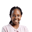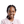Trusted answers to developer questions
Trusted Answers to Developer Questions

Related Tags

linear algebra
python
communitycreator

# What is scipy.linalg.expm() in Python?Joy Kareko

Grokking Modern System Design Interview for Engineers & Managers

Ace your System Design Interview and take your career to the next level. Learn to handle the design of applications like Netflix, Quora, Facebook, Uber, and many more in a 45-min interview. Learn the RESHADED framework for architecting web-scale applications by determining requirements, constraints, and assumptions before diving into a step-by-step design process.

### Introduction

Linear Algebra is a branch of mathematics concerned with linear equations and other mathematical computations. Scipy is a Python library that is useful for such mathematical and scientific computations.

The expm() function computes and returns the exponential of a matrix.

### Syntax

scipy.linalg.expm(A)



### Parameters

A: This is the matrix whose exponential we want to obtainin.

### Return value

The function returns the exponential of the matrix A.

### Example

import scipyimport numpy as npfrom scipy.linalg import expmA = np.matrix([[0.234,0.567], [1.249,2.367]])print(A)B = expm(A)print('-------------------')print(B)
Run to see the results! Try changing input arguments to experiment further.

### Explanation

• Lines 1–4: We import the packages numpy, scipy, and expm.
• Line 6: We declare a matrix A.
• Line 10: We compute the exponential of matrix A.
• Lines 8 and 14: We print the resulting matrices.

RELATED TAGS

linear algebra
python
communitycreator

CONTRIBUTORJoy Kareko

Grokking Modern System Design Interview for Engineers & Managers

Ace your System Design Interview and take your career to the next level. Learn to handle the design of applications like Netflix, Quora, Facebook, Uber, and many more in a 45-min interview. Learn the RESHADED framework for architecting web-scale applications by determining requirements, constraints, and assumptions before diving into a step-by-step design process.

Keep Exploring

Learn in-demand tech skills in half the time